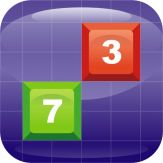Español

Cada día ofrecemos un programa licenciado GRATIS que de otra manera tendrías que comprar!\$0.99
EXPIRÓ

# iPhone Giveaway of the Day - Digtris - Digital Tetris

Digtris is a mathematical game similar to Tetris.
\$0.99 EXPIRÓ

Esta oferta de regalo ha expirado. Digtris - Digital Tetris ya está disponible.

Digtris is a mathematical game similar to Tetris. The 6х9 playing field is being filled with cubes with digits from 1 to 9. Your task is to liberate the field from the cubes that disappear if the sum of the adjacent cubes is equal to or multiple of 10.

The game has 7 modes:

2 figures - the cubes disappear if the sum of 2 cubes is equal to 10 horizontally or vertically..
3 figures - the cubes disappear if the sum of 3 cubes is multiple of 10 vertically or horizontally.
4 figures - the cubes disappear if the sum of 4 cubes is multiple of 10 vertically or horizontally.
Line - the cubes disappear if the sum of any quantity of the cubes is divisible by 10 horizontally.
Square 2x2 - the cubes disappear if their amount in any 2x2 square is multiple of 10.
Square 3x3 - the cubes disappear if their amount in any 3x3 square is multiple of 10.
Rectangle 2х3 - the cubes disappear if their amount in any 2x3 rectangle is multiple of 10.

Alina Bagrova

Games

1.1

35.26 MB

4+

### Idiomas:

English, Russian